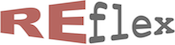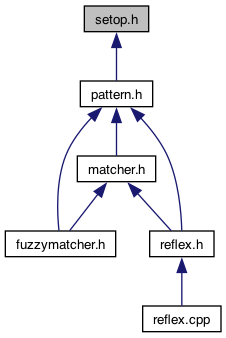setop.h File Reference updated Sun Aug 21 2022 by Robert van Engelen
setop.h File Reference

RE/flex operations on STL containers and sets. More...

This graph shows which files directly or indirectly include this file:## Classes

struct  reflex::lazy_intersection< S1, S2 >
Intersection of two ordered sets, with an iterator to get elements lazely. More...

struct  reflex::lazy_intersection< S1, S2 >::iterator
Iterator to lazely get elements of a set intersection. More...

struct  reflex::lazy_union< S1, S2 >
Union of two ordered sets, with an iterator to get elements lazely. More...

struct  reflex::lazy_union< S1, S2 >::iterator
Iterator to lazely get elements of a set union. More...

reflex

## Functions

template<typename S1 , typename S2 >
bool reflex::is_disjoint (const S1 &s1, const S2 &s2)
Check if sets `s1` and `s2` are disjoint. More...

template<typename T , typename S >
bool reflex::is_in_set (const T &x, const S &s)
Check if value `x` is in set `s`. More...

template<typename S1 , typename S2 >
bool reflex::is_subset (const S1 &s1, const S2 &s2)
Check if set `s1` is a subset of set `s2`. More...

template<typename S1 , typename S2 >
void reflex::set_insert (S1 &s1, const S2 &s2)
Insert set `s2` into set `s1`. More...

template<typename S , typename E >
void reflex::set_add (S &s, const E &e)
Insert element `e` into set `s`. More...

template<typename S1 , typename S2 >
void reflex::set_delete (S1 &s1, const S2 &s2)
Delete elements of set `s2` from set `s1`. More...

template<typename S , typename E >
void reflex::set_erase (S &s, const E &e)
Remove element `e` from set `s` when present. More...

## Detailed Description

RE/flex operations on STL containers and sets.

## Usage

`reflex::is_disjoint<S1,S2>(S1 s1, S2 s2)` is true if `s1` and `s2` are disjoint sets.

`reflex::is_subset<S1,S2>(S1 s1, S2 s2)` is true if `s1` is a subset of `s2`.

`reflex::is_in_set<T,S>(T x, S s)` is true if `x` is in `s`.

`reflex::set_insert<S1,S2>(S1 s1, S2 s2)` inserts elements of `s2` into `s1`.

`reflex::set_delete<S1,S2>(S1 s1, S2 s2)` deletes elements of `s2` from `s1`.

`reflex::lazy_intersection<S1,S2>(S1 s1, S2 s2)` is a structure with an iterator over elements that are in `s1` and in `s2`.

`reflex::lazy_union<S1,S2>(S1 s1, S2 s2)` is a structure with an iterator over elements that are in `s1` or in `s2`.

The rationale for using `reflex::lazy_intersection` and `reflex::lazy_union` is to save memory when the results do not need to be stored in a set, since the elements are lazely produced by an iterator.

## Example

std::set<int> s1;
s1.insert(1);
assert(reflex::is_in_set(1, s1) == true);
std::set<int> s2;
s2.insert(1);
s2.insert(2);
assert(reflex::is_disjoint(s1, s2) == false);
assert(reflex::is_subset(s1, s2) == true);
reflex::lazy_union< std::set<int>,std::set<int> > U(s1, s2);
for (reflex::lazy_union< std::set<int>,std::set<int> >::iterator i = U.begin(); i != U.end(); ++i)
std::cout << *i << std::endl; // prints 1 and 2
reflex::lazy_intersection< std::set<int>,std::set<int> > I(s1, s2);
for (reflex::lazy_intersection< std::set<int>,std::set<int> >::iterator i = I.begin(); i != I.end(); ++i)
std::cout << *i << std::endl; // prints 1